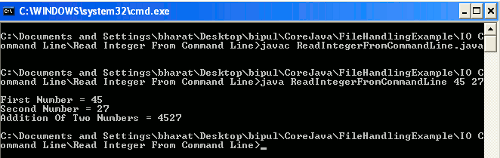Tutorials

How To Read Integer From Command Line In Java

In this section we will discuss about how an integer can be read through the command line.

In this section we will discuss about how an integer can be read through the command line.

How To Read Integer From Command Line In Java

In this section we will discuss about how an integer can be read through the command line.

In Java all the inputs are treated as a String. So, input for other than the String is required to parse into its type by wrapping their respective wrapper class available in Java. In Java their are various wrapper classes of primitive data types are provided. These are as follows :

 Primitive Type Wrapper Class boolean Boolean byte Byte char Character float Float int Integer long Long short Short

parseXXX() method of these wrapper class is required to parse these primitive data type.

Note : In case of char we can use charAt() method discussed in example with comment.

Example

Here I am giving a simple example which will demonstrate you about how to take integer value from the command line. In this example I have created a class named ReadIntegerFromCommandLine.java where I have tried to convert the command line argument input by the keyboard to the Integer. Then get the addition of these two values.

Source Code

{
public static void main(String args[])
{
if(args.length > 0)
{
try
{

// For taking char input
// char c = args.charAt(0);
// For Number
int firstNum = Integer.parseInt(args);
int secNum = Integer.parseInt(args);
int sum = firstNum+secNum;

/*
String firstNum = args;
String secNum = args;
String sum = firstNum+secNum;
*/

System.out.println("First Number = "+firstNum);
System.out.println("Second Number = "+secNum);
System.out.println("Addition Of Two Numbers = "+sum);
}
catch(NumberFormatException nfe)
{
System.out.println("Argument/s must be the integer value");
}
}
}
}

Output

When you will execute the above example by giving the rigth arguments i.e. the integer numberic value then the output will be as follows :But if in the program you will not parse the primitive value and give the argument as numeric value then it will displays the number as you have provided in the argument but the '+' operator will not function as mathematically. It will simply concatenate these two inputs as given in the output below :